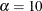# The FREQ Procedure

### Example 3.4 Binomial Proportions

In this example, PROC FREQ computes binomial proportions, confidence limits, and tests. The example uses the eye and hair color data from Example 3.1. By default, PROC FREQ computes the binomial proportion as the proportion of observations in the first level of the one-way table. You can designate a different level by using the LEVEL= binomial-option.

The following PROC FREQ statements compute the proportion of children with brown eyes (from the data set in Example 3.1) and test the null hypothesis that the population proportion equals 50%. These statements also compute an equivalence test for the proportion of children with fair hair.

The first TABLES statement requests a one-way frequency table for the variable Eyes. The BINOMIAL option requests the binomial proportion, confidence limits, and test. PROC FREQ computes the proportion with Eyes = 'brown', which is the first level displayed in the table. The AC, WILSON, and EXACT binomial-options request the following confidence limits types: Agresti-Coull, Wilson (score), and exact (Clopper-Pearson). By default, PROC FREQ provides Wald and exact (Clopper-Pearson) confidence limits for the binomial proportion. The BINOMIAL option also produces an asymptotic Wald test that the proportion is 0.5. You can specify a different test proportion in the P= binomial-option. The ALPHA=0.1 option specifies that%, which produces 90% confidence limits.

The second TABLES statement requests a one-way frequency table for the variable Hair. The BINOMIAL option requests the proportion for the first level, Hair = 'fair'. The EQUIV binomial-option requests an equivalence test for the binomial proportion. The P=.28 option specifies 0.28 as the null hypothesis proportion, and the MARGIN=.1 option specifies 0.1 as the equivalence test margin.

proc freq data=Color order=freq;
tables Eyes / binomial(ac wilson exact) alpha=.1;
tables Hair / binomial(equiv p=.28 margin=.1);
weight Count;
title 'Hair and Eye Color of European Children';
run;


Output 3.4.1 displays the results for eye color, and Output 3.4.2 displays the results for hair color.

Output 3.4.1: Binomial Proportion for Eye Color

 Hair and Eye Color of European Children

The FREQ Procedure

Eye Color
Eyes Frequency Percent Cumulative
Frequency
Cumulative
Percent
brown 341 44.75 341 44.75
blue 222 29.13 563 73.88
green 199 26.12 762 100.00

Binomial Proportion
Eyes = brown
Proportion 0.4475
ASE 0.0180

Confidence Limits for the Binomial Proportion
Proportion = 0.4475
Type 90% Confidence Limits
Agresti-Coull 0.4181 0.4773
Clopper-Pearson (Exact) 0.4174 0.4779
Wilson 0.4181 0.4773

Test of H0: Proportion = 0.5
ASE under H0 0.0181
Z -2.8981
One-sided Pr < Z 0.0019
Two-sided Pr > |Z| 0.0038

The frequency table in Output 3.4.1 displays the values of Eyes in order of descending frequency count. PROC FREQ computes the proportion of children in the first level displayed in the frequency table, Eyes = 'brown'. Output 3.4.1 displays the binomial proportion confidence limits and test. The confidence limits are 90% confidence limits. If you do not specify the ALPHA= option, PROC FREQ computes 95% confidence limits by default. Because the value of Z is less than 0, PROC FREQ displays the a left-sided p-value (0.0019). This small p-value supports the alternative hypothesis that the true value of the proportion of children with brown eyes is less than 50%.

Output 3.4.2 displays the equivalence test results produced by the second TABLES statement. The null hypothesis proportion is 0.28 and the equivalence margins are –0.1 and 0.1, which yield equivalence limits of 0.18 and 0.38. PROC FREQ provides two one-sided tests (TOST) for equivalence. The small p-value indicates rejection of the null hypothesis in favor of the alternative that the proportion is equivalent to the null value.

Output 3.4.2: Binomial Proportion for Hair Color

Hair Color
Hair Frequency Percent Cumulative
Frequency
Cumulative
Percent
fair 228 29.92 228 29.92
medium 217 28.48 445 58.40
dark 182 23.88 627 82.28
red 113 14.83 740 97.11
black 22 2.89 762 100.00

Equivalence Analysis
H0: P - p0 <= Lower Margin or >= Upper Margin
Ha: Lower Margin < P - p0 < Upper Margin
p0 = 0.28   Lower Margin = -0.1   Upper Margin = 0.1
Proportion ASE (Sample)
0.2992 0.0166

Two One-Sided Tests (TOST)
Test Z P-Value
Lower Margin 7.1865 Pr > Z <.0001
Upper Margin -4.8701 Pr < Z <.0001
Overall     <.0001

Equivalence Limits 90% Confidence Limits
0.1800 0.3800 0.2719 0.3265